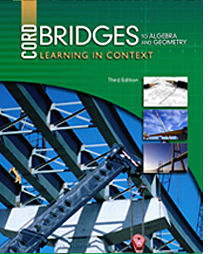# Bridges to Algebra and Geometry - 3rd Edition

## Chapter 11: Measurement

Some links are repeated for use with more than one lesson.

### 11.1  Similar Figures

http://www.regentsprep.org/Regents/math/geometry/GP11/LMoresimilar.htm
NEW This page gives the requirements for figures to be considered similar.

http://www.mathsisfun.com/geometry/similar.html
NEW This page defines similar figures and introduces the geometric transformations that yield similar figures, including practice problems.

http://www.math.com/school/subject1/lessons/S1U2L4GL.html
This site defines similar figures.

### 11.2  Dilations

http://www.regentsprep.org/Regents/math/geometry/GT3/Ldilate.htm
NEW This site presents an intuitive understanding of dilations. More advanced content is available, if desired.

http://www.mathwarehouse.com/transformations/dilations/dilations-in-math.php

http://www.mathsisfun.com/geometry/resizing.html
NEW This page presents the concept of dilations as “resizing,” including practice problems.

### 11.3  Scale Drawings and Maps

http://www.wikihow.com/Draw-a-Floor-Plan-to-Scale
NEW This page describes the steps to construct a scaled floor plan.

### 11.5  Perimeter and Area of Polygons

http://www.mathsisfun.com/area.html
NEW This page provides the area formulas for many basic polygons, and tips for working with irregular shapes, including practice problems.

http://www.regentsprep.org/regents/math/algebra/as1/indexAS1.htm
NEW This site includes links to several pages discussing area calculations, including hints and practice problems.

### 11.6  Perimeter and Area of Similar Polygons

http://www.regentsprep.org/Regents/math/geometry/GP11/LMoresimilar.htm
NEW This page summarizes the relationship on perimeter, area, and more aspects of similar figures.

http://www.mathwarehouse.com/geometry/similar/triangles/area-and-perimeter-of-similar-triangles.php
NEW This page discusses the area and perimeter of similar triangles, with practice problems.

### 11.7  Circles

http://www.mathsisfun.com/geometry/circle.html
NEW This page presents the parts and features of circles, including practice problems.

http://teachingmath.info/pie.htm
NEW This page derives the value and use of pi in circle calculations with an unusual approach, perhaps making it easier to remember.

http://www.piday.org
NEW This site is devoted to interesting facts and knowledge of pi, including a listing of one million digits of pi.

http://www.angio.net/pi
NEW This page includes a very fast search engine to find sequences of digits (e.g., your birthdate) in the value of pi, and links to files with many digits of pi.